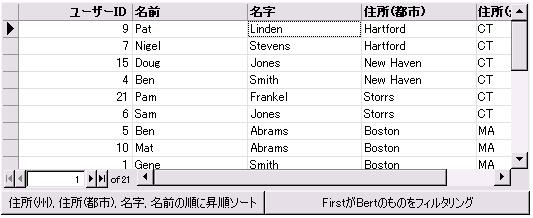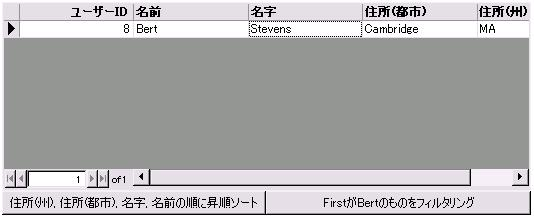# RecordViewを用いたデータのソート・抽出

RecordView は、基になるソース RecordSet に含まれているデータに効率よくアクセスするための基本的な手段です。

RecordViewを使用することで、フィルタリングで抽出したデータだけを照会することが出来ます。

アプリケーションロジックを適用してデータのソートを行ったり、
フィルタリングを実施することが出来ます。
RecordViewを用いて特定のデータを抽出したからといって、

RecordViewのサンプルコードは以下の通りです。

 {curl 6.0 applet}{curl-file-attributes character-encoding = “shift-jis”} {let staff:RecordSet =     {RecordSet        {RecordFields            {RecordField                 “id”, caption = “ユーザーID”, domain = int,                 index-type = RecordFieldIndexType.unique            },            {RecordField “First”, domain = String, caption = “名前”},            {RecordField “Last”, domain = String , caption = “名字”},            {RecordField “City”, domain = String , caption = “住所(都市)”},            {RecordField “State”, domain = String, caption = “住所(州)”}        },        {RecordData id = 1, First = “Gene”, Last = “Smith”, City = “Boston”, State = “MA”},        {RecordData id = 2, First = “Fred”, Last = “Smith”, City = “Cambridge”, State = “MA”},        {RecordData id = 3, First = “Mike”, Last = “Smith”, City = “Keene”, State = “NH”},        {RecordData id = 4, First = “Ben”, Last = “Smith”, City = “New Haven”, State = “CT”},        {RecordData id = 5, First = “Ben”, Last = “Abrams”, City = “Boston”, State = “MA”},        {RecordData id = 6, First = “Sam”, Last = “Jones”, City = “Storrs”, State = “CT”},        {RecordData id = 7, First = “Nigel”, Last = “Stevens”, City = “Hartford”, State = “CT”},        {RecordData id = 8, First = “Bert”, Last = “Stevens”, City = “Cambridge”, State = “MA”},        {RecordData id = 9, First = “Pat”, Last = “Linden”, City = “Hartford”, State = “CT”},        {RecordData id = 10, First = “Mat”, Last = “Abrams”, City = “Boston”, State = “MA”},        {RecordData id = 11, First = “John”, Last = “Rogers”, City = “Cambridge”, State = “MA”},        {RecordData id = 12, First = “Dan”, Last = “Abrams”, City = “Keene”, State = “NH”},        {RecordData id = 13, First = “Chris”, Last = “Abrams”, City = “Cambridge”, State = “MA”},        {RecordData id = 14, First = “Glenn”, Last = “Abrams”, City = “Keene”, State = “NH”},        {RecordData id = 15, First = “Doug”, Last = “Jones”, City = “New Haven”, State = “CT”},        {RecordData id = 16, First = “Susan”, Last = “Rogers”, City = “Concord”, State = “NH”},        {RecordData id = 17, First = “Joan”, Last = “Smith”, City = “Concord”, State = “MA”},        {RecordData id = 18, First = “Tom”, Last = “Frankel”, City = “Keene”, State = “NH”},        {RecordData id = 19, First = “Sarah”, Last = “Frankel”, City = “Concord”, State = “NH”},        {RecordData id = 20, First = “John”, Last = “Jones”, City = “Keene”, State = “NH”},        {RecordData id = 21, First = “Pam”, Last = “Frankel”, City = “Storrs”, State = “CT”}    }}{let rv:RecordView =     {RecordView staff}}{let rg:RecordGrid =     {RecordGrid        record-source = rv, height = 5cm, width = 14cm    }}{value     {VBox        rg,        {HBox            {CommandButton                 width = {make-elastic},                label = “住所(州), 住所(都市), 名字, 名前の順に昇順ソート”,                {on Action do                    set rv.sort = “State, City, Last, First”                }            },            {CommandButton                 width = {make-elastic},                label = “FirstがBertのものをフィルタリング”,                {on Action do                    set rv.filter = {RecordData First = “Bert”}                }            }        }    }        }

このサンプルを実行すると以下のような結果が得られます。ただし、RecordViewを作成したからといって、
RecordSetに含まれる各レコードが個別にコピーされた訳ではありません。
RecordViewで抽出したレコードに格納されている値を直接書き換えてしまうと、
RecordSetに登録されている値も変更されてしまうので、注意が必要です。

また、レコードのステータスを更新するRecordViewの以下のメソッドは、

そのため、RecordSet側でデータが更新されていた場合は、
RecordViewの方でデータの更新を行っていなくとも

○RecordView.commit
○RecordView.revert
○RecordView.load
○RecordView.load-state
○RecordView.pending-update?